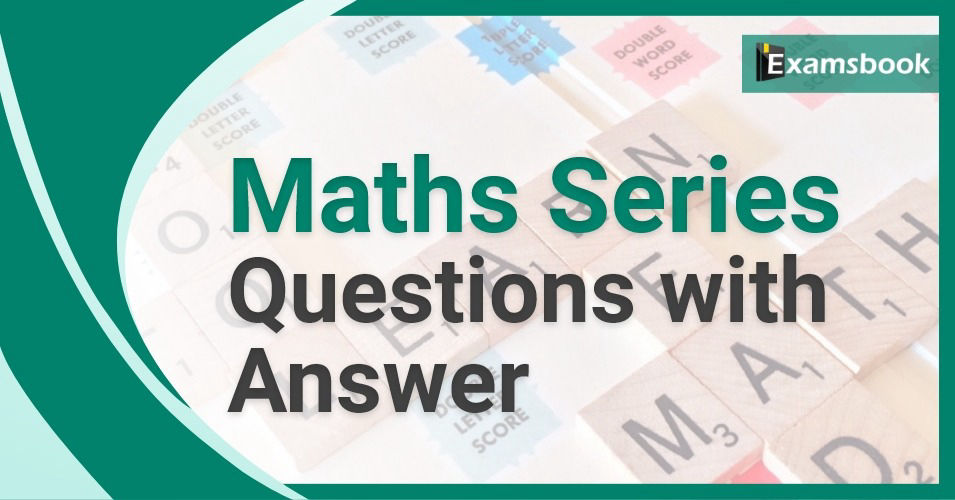• Save

# Maths Series Questions with Answers

Vikram SinghLast year 71.8K ViewsIn almost all competitive examinations conducted by the central and state government, are asked questions related to the subject of Mathematics. Here are selective Maths questions and answers for SSC, Bank, RRB and other government exams. Students have to need special knowledge of maths questions

So, I have provided questions on maths questions to the students who are preparing for competitive examinations. Also with the help of this article, you can continuously practice the questions given for better results in the exam.

## Mathematical Series Questions for Competitive Exams

Q. If a number multiplied by 25% of itself gives a number which is 200% more than the number, then the number is –

a. 12
b. 16
c. 35
d. 24

Q. The value of an article depreciates every year at the rate of 10% of its value. If the present value of the article is Rs.729, then its worth 3 years ago was-

a. Rs.1250
b. Rs.1000
c. Rs.1125
d. Rs.1200

Q. The price of onions has been increased by 50%. In order to keep the expenditure on onions the same the percentage of reduction in consumption has to be-

a. 50%
b. 33 1/3%
c. 33%
d. 30%

Q. If a regular polygon has each of its angles equal to 3/5 times of two right angles, then the number of side is-

a. 3
b. 5
c. 6
d. 8

Ask me in the comment section, If you face any problem while solving maths questions. Visit next page for more maths problems.

### Vikram Singh

Providing knowledgable questions of Reasoning and Aptitude for the competitive exams.